# How to use OFFSET Function in Excel

Syntax of OFFSET

=OFFSET(reference,rows, columns, [height], [width])

The OFFSET function returns reference of cell from given offset (row and column) of given reference.

For example, if you give reference of B4, offset row 0, and offset column 1 to OFFSET function, then value from C4 will be returned. Confusing? Lets have some more examples. Last example of OFFSET function tells how to SUM dynamically.

#### OFFSET Function to Get value From Right and Left of a Cell

We have this table.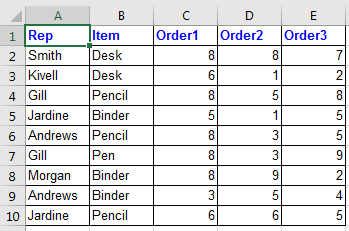Now if I want to get value from 2 cell right to B2 (which means D2). I will write this OFFSET formula:

=OFFSET(B2,0,2)

OFFSET function will move 2 cell to right of cell B4 and return its value. See Image below

If I want to get value from 1 cell left to B2 (which means A2). I will write this OFFSET formula:

=OFFSET(B2,0,-1)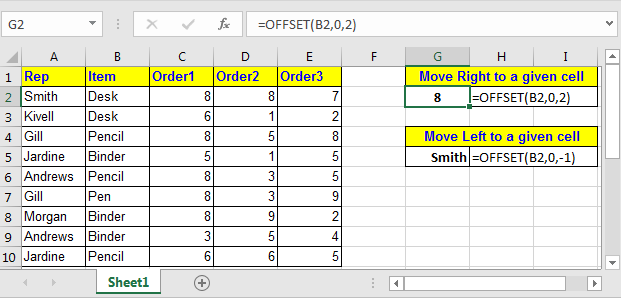#### OFFSET Function to Get value From Below and Above of a Cell

So again in the above table, if I want to get value from 2 cell belove to B3 (which means B5). I will write this OFFSET formula:

=OFFSET(B2,2,0)

If I want to get value from 1 cell left to B2 (which means A2). I will write this OFFSET formula:

=OFFSET(B2,-2,0)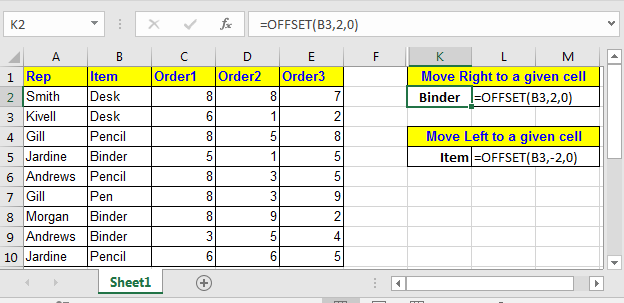• Pro Tip: Treat a OFFSET as graph pointer where Reference is origin and row and column are x and y axis.

#### OFFSET Function to Sum Range Dynamically

Let's see this example.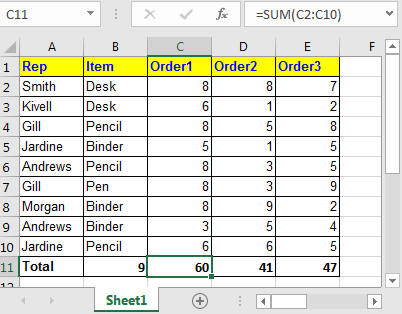In the above table, the total row is at the end of the table. Over the time you will add rows to this table. Then you’ll need to change sum range in the formula. Here we can take help of the OFFSET function to sum dynamically. Currently, in cell C11, we have =SUM(C2:C10). Replace this with below formula.

=SUM(C2:OFFSET(C11,-1,0))

This formula just takes the first data row and then sums the range above the total row.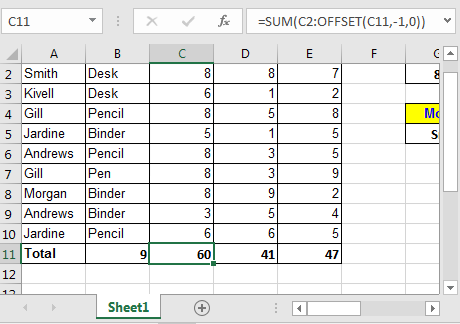#### Offset For Getting Array

OFFSET function has two optional arguments, [height] and [width]. Do not take them for granted. When OFFSET function is provided [height] and [width] arguments, it returns a two-dimensional array. If only one of them is passed as an argument it returns on a dimensional array of values.

If you type in this formula in any cell :

=SUM(OFFSET(C1,1,0,9,3))

It will sum values in range starting from C2 to 9 rows below and 3 columns right.

OFFSET(C1,1,0,9,3) : This will translate to {8,8,7;6,1,2;8,5,8;5,1,5;8,3,5;8,3,9;8,9,2;3,5,4;6,6,5}.So in conclusion, offset is very useful function of excel that can help you solve many strangled threads. Let me know how you use OFFSET function in your formula and where it helped you most.

Related Articles:

How to Get Every Nth Value From A list

How to Get Every Nth Value From A list

How To Look Up Address in Excel

Popular Articles:

50 Excel Shortcuts to Increase Your Productivity

How to use the VLOOKUP Function in Excel

How to use the COUNTIF function in Excel 2016

How to Use SUMIF Function in Excel

Terms and Conditions of use

The applications/code on this site are distributed as is and without warranties or liability. In no event shall the owner of the copyrights, or the authors of the applications/code be liable for any loss of profit, any problems or any damage resulting from the use or evaluation of the applications/code.